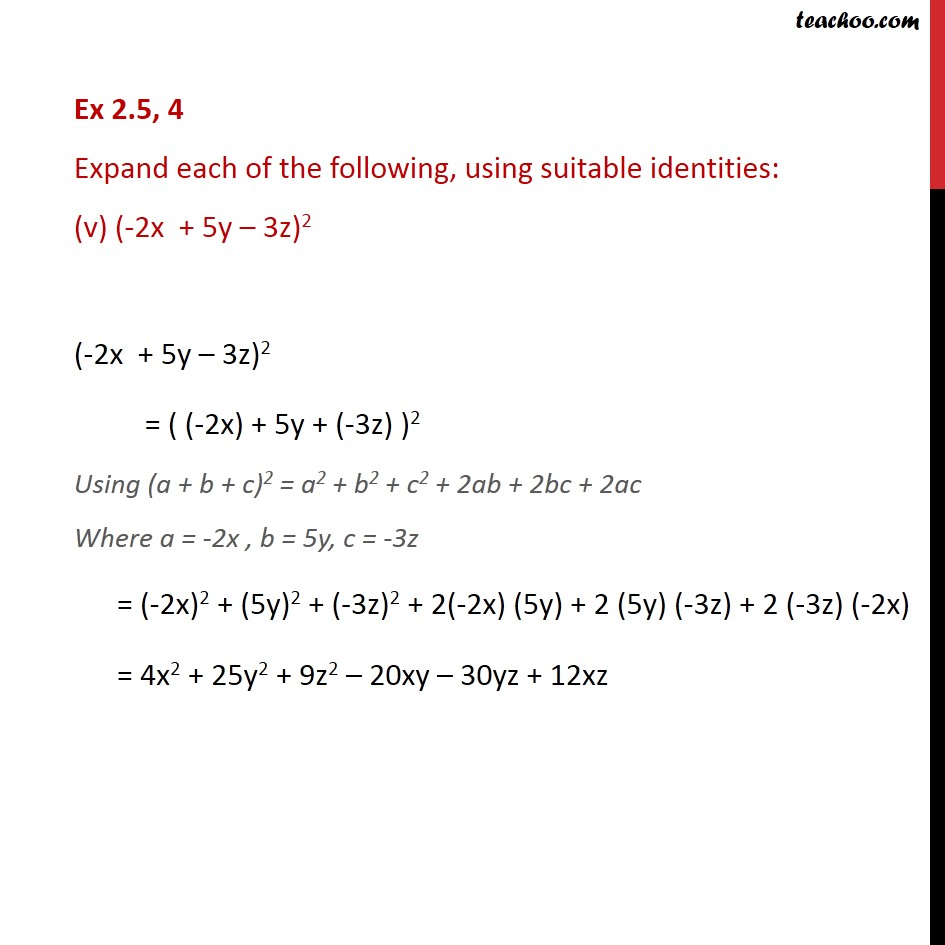Ex 2.4

Chapter 2 Class 9 Polynomials
Serial order wiseLearn in your speed, with individual attention - Teachoo Maths 1-on-1 Class

### Transcript

Ex 2.4, 4 Expand each of the following, using suitable identities: (v) (-2x + 5y 3z)2 (-2x + 5y 3z)2 = ( (-2x) + 5y + (-3z) )2 Using (a + b + c)2 = a2 + b2 + c2 + 2ab + 2bc + 2ac Where a = -2x , b = 5y, c = -3z = (-2x)2 + (5y)2 + (-3z)2 + 2(-2x) (5y) + 2 (5y) (-3z) + 2 (-3z) (-2x) = 4x2 + 25y2 + 9z2 20xy 30yz + 12xz